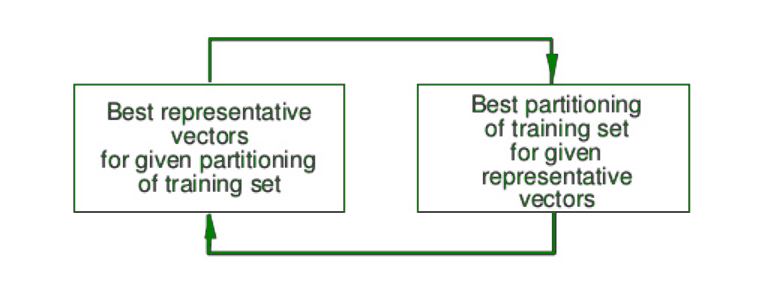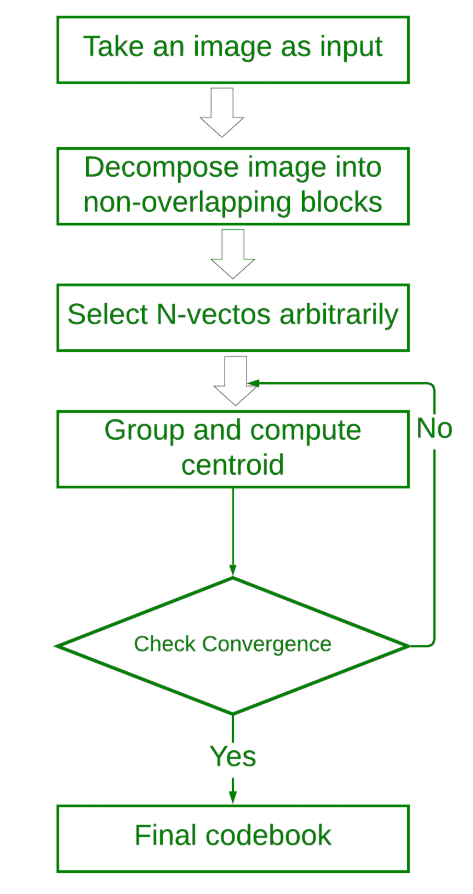Related Articles

# Linde-Buzo-Gray (LBG) Algorithm

• Last Updated : 08 Jan, 2020

Linde-Buzo-Gray (LBG) Algorithm is used for designing of Codebook efficiently which has minimum distortion and error.

LBG algorithm was proposed by Yoseph Linde, Andres Buzo and Robert M. Gray in 1980. It is the most common algorithm for Code Generation that generates a codebook with minimum error from a training set.Training sets are a set of vectors that are derived from image vectors. The code vectors must minimize the distortion.

LBG algorithm assumes the codeword length to be fixed.

It is an iterative procedure and the basic idea is to divide the group of training vectors and use it to find the most representative vector from one group. These representative vectors from each group are gathered to form the codebook.

Procedure for LBG Algorithm:

1. Divide the given image into blocks, so that each block appears as a d-dimensional vector.
For example, if image size is 712×712 and block size is 4×4, then the number of blocks is equal to (712*712)/(4*4)=31684.
And each block is a 16 dimension vector.
2. Initial codebook is chosen randomly.
3. The initial chosen codebook is set as the centroid and the other vectors are grouped according to the nearest distance with the centroid similar to the k-mean clustering.
4. Find the new centroid of every group to get a new codebook iterative. Repeat the steps 2 & 3 till the convergence of the centroid of every group.

Flow Diagram of LBG Algorithm:My Personal Notes arrow_drop_up